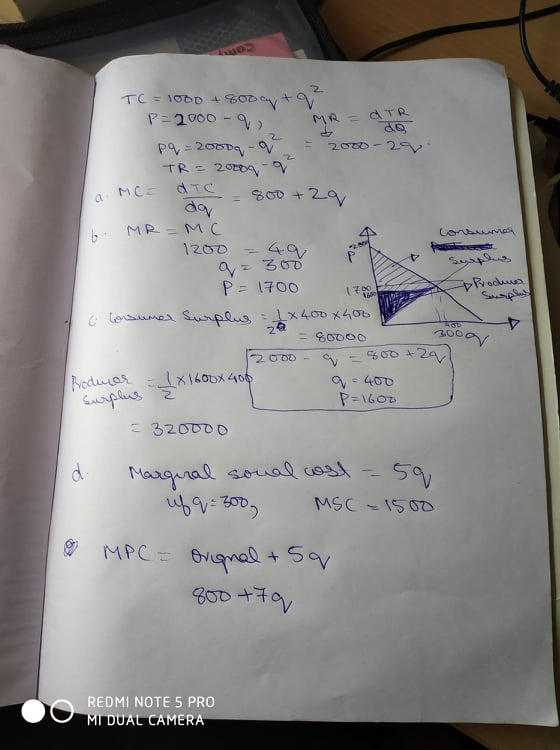Homework Help Question & Answers

# Suppose that the Chengdu Style restaurant sells an amount q of food; the total cost function for ...

Suppose that the Chengdu Style restaurant sells an amount q of food; the total cost function for Chengdu is given by:   TC = 1000 + 800q + q2.
The quantity (q) demanded for Chengdu Style food is very high. Suppose that it is given by:

q = 2000 − pwhere p is the price of food sold at Chengdu.

(a) What is the marginal private cost of Chengdu Style?

(b) Suppose Chengdu’s clients have several other restaurants to go to in and around Davis such that Chengdu faces a perfectly competitive market. If Chengdu maximizes profits by choice of quantity q, what is the market equilibrium quantity and price?

(c) What is the consumer surplus and producer surplus?

(d) Suppose that Chengdu Style utilizes palm oil in their cooking which causes damages to the society equivalent to 5q per unit of food produced. What is the marginal private cost of Chengdu Style? What is the marginal social cost of Chengdu Style?

(e) What is the social optimal level of Chengdu Style’s food production and the social optimal price?

#### Homework Answers

ReportAnswer #1Add Homework Help Answer
##### Add Answer of: Suppose that the Chengdu Style restaurant sells an amount q of food; the total cost function for ...
Your Answer: Your Name: What's your source?
Not the answer you're looking for? Ask your own homework help question. Our experts will answer your question WITHIN MINUTES for Free.
More Homework Help Questions Additional questions in this topic.

• #### The overall (weighted average) cost of capital is composed of a weighted average of : a)The cost of common equity and the cost of debt b)The cost of common equity and the cost of preferred stock c)The cost of preferred stock and the cost of debt d)The cos

Need Online Homework Help?

Get FREE EXPERT Answers
WITHIN MINUTES
Related Questions